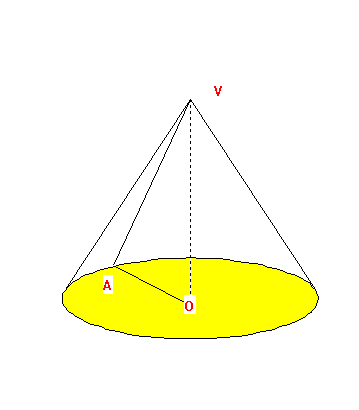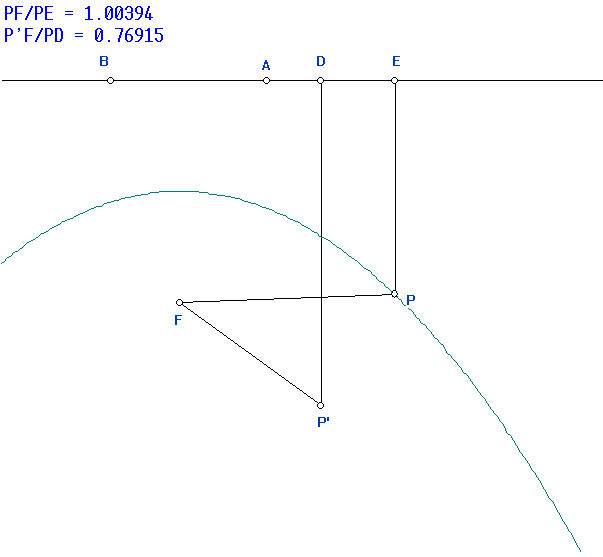"Conics" [VIDEO #628].

• This video outlines two separate ways to understand the conics as a family of curves using the traditional "Euclidean" views of the conics starting with a cross section of a cone. Two ways to characterize the conics were treated in the video -
• i....related to 2 foci (ellipse and hyperbola) or 1 focus (parabola),
ii...related to a focus and directrix which was also related to eccentricity.
Using two spheres tangent at two focii (F1 and F2) to the sectioning plane that also touch the sides of the cone, the ellipse is characterized by the distance from a point on the ellipse P having the sum of the distances PF1 + PF2 a constant,
• Consider a circle in a plane and a single point in space not on that plane (the vertex or apex). This will determine a cone, made from the lines passing through vertex and points on the circle.• Usually we think of the point lying on the line perpendicular to the center of the circle and only the part of the cone determined by line segments from the vertex and the points on the circle. However, the cone does extend indefinitely beyond the circle and the vertex as the lines extend indefinitely. When we cut a cone with a plane not passing through the vertex we determine a curve in the plane, described as the "conic section." [If the plane passes through the vertex the interesection can be a point (the vertex itself), a single line element of the cone, or a pair of intersecting lines that meet at the vertex.] These are circles, ellipses, parabolas, and hyperbolas.
while the hyperbola has the magnitude (absolute value) of the difference of these lengths |PF1-PF2| a constant.The parabola which in a sense lies between the ellipses and hyperbolae does not fit into these descriptions using two focii. The film explains and visualizes these relations.
• Again using the spheres and choosing one of these, consider the plane through the sphere at the circle where the sphere touched the cone. This plane meets the sectioning plane on a line, called the directrix, d. The film shows that for any conic section a point P on the conic is characterized by considering the ratio of PF1 to the distance from that point to the directrix, Pd . This ratio (called the eccentricity of the conic, e) is a constant for each conic section, in fact the ratio of the sines of the angle between the sectioning plane and the circle's plane and the angle between the cone and the circle's plane.• The following table summarizes the results related to this ratio. It is fully explained in the video.

 ratio = e Conic e = 0 circle 0 < e < 1 ellipse e = 1 parabola e > 1 hyperbola
• Notice that from the ideas of projection from the vertex of the cone, any of the conic sections could be the shadow cast by the circle on a sectioning plane.